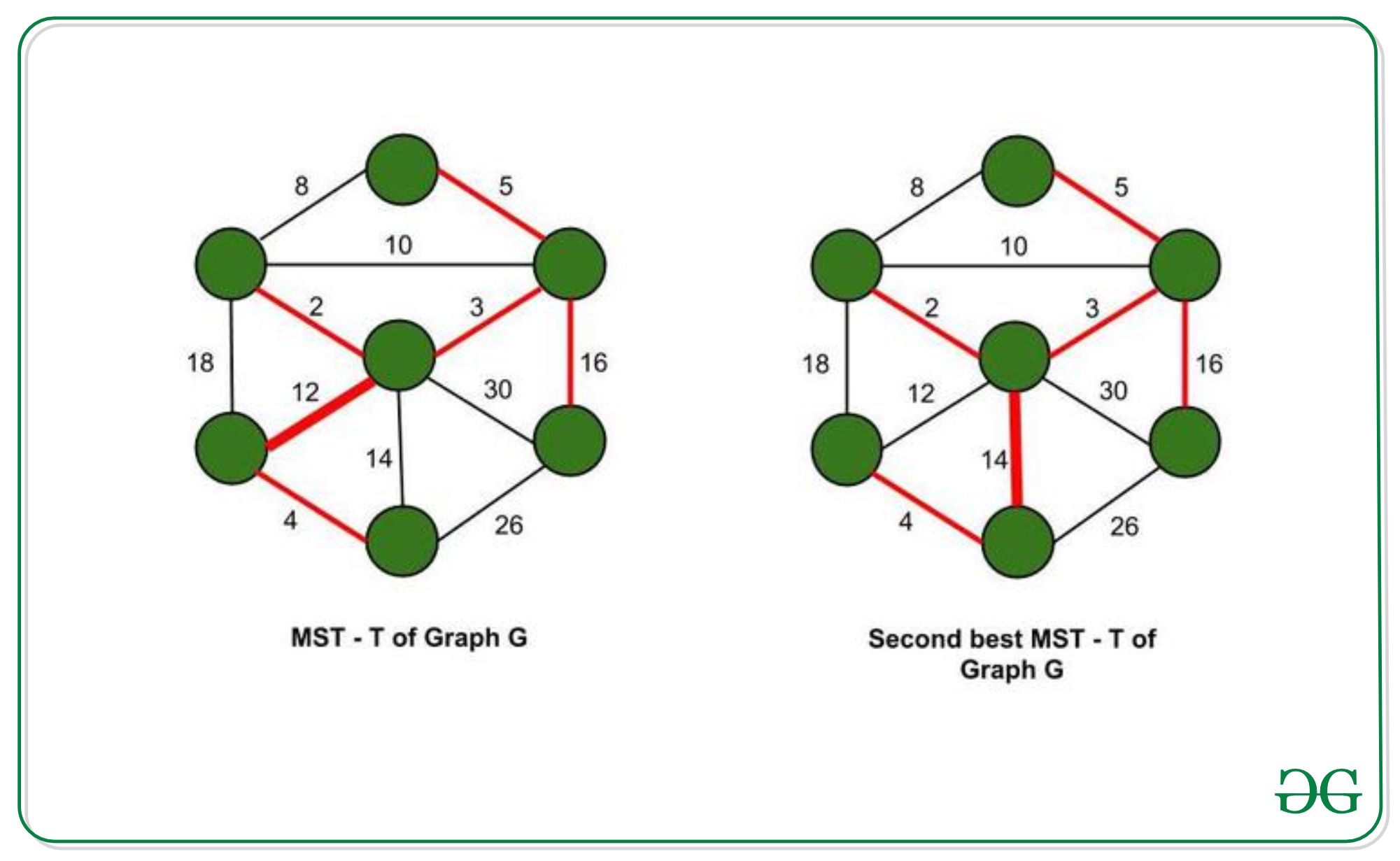# Second Best Minimum Spanning Tree

• Difficulty Level : Hard
• Last Updated : 23 Jul, 2021

Prerequisites Graph, Spanning tree, Disjoint Set (Union – Find).

A minimum spanning tree (MST) T, for a given graph G, spans over all vertices of a given graph and has minimum weight sum of all edges, out of all the possible spanning trees.

Second best MST, T’, is a spanning tree with the second minimum weight sum of all edges, out of all spanning trees of graph G.

T and T’ differ by only one edge replacement. So, we should find an edge enew which is not in T and replace it with an edge in T (say eold) such that T’ = T union {enew} – {eold} is a spanning tree and weight difference of (enew – eold) is minimum (enew, eold are edges in the graph G).Using Kruskal’s Algorithm

• Use Kruskal’s algorithm to find MST T of graph G. Remove a single edge from it and replace it with another to obtain T’.
• Sort the edges in O(ElogE) time (E-no.of edges) and find MST using Kruskal’s algorithm in O(E) time (No.of edges in MST = V-1 where V = no.of vertices in the graph G).
• For each edge in MST, temporarily exclude it from the edge list (so that we cannot choose it).
• Then, try to find MST in O(E) using the remaining edges. (no need to sort again)
• Repeat the above for all edges in MST and take the best one. (with 2nd minimum weight sum). Thus, we obtained  T’ – second-best MST.
• Overall Time Complexity – O(ElogE + E +VE) = O(VE)

Below is the implementation of the above approach:

## C++

 `// C++ implementation to find the``// second best MST`` ` `#include ``using` `namespace` `std;`` ` `// used to implement union-find algorithm``int` `parent;`` ` `// to keep track of edges in MST``vector<``int``> present;`` ` `// to keep track of number of edges``// in spanning trees other than the MST``int` `edg;`` ` `// a structure to represent a``// weighted edge in graph``struct` `edge {``    ``int` `src, dest, weight;``} edges;``// array edges is of type edge.`` ` `// Compare two edges according``// to their weights.``// Used in sort() for sorting``// an array of edges``bool` `cmp(edge x, edge y)``{``    ``return` `x.weight < y.weight;``}`` ` `// initialising the array -``// each vertex is its own parent``// initially``void` `initialise(``int` `n)``{``    ``// 1-indexed``    ``for` `(``int` `i = 1; i <= n; i++)``        ``parent[i] = i;``}`` ` `// Implementing the union-find algorithm``int` `find(``int` `x)``{``    ``if` `(parent[x] == x)``        ``return` `x;``    ``return` `parent[x] = find(parent[x]);``}`` ` `// Function to find the union``// for the Minimum spanning Tree``int` `union1(``int` `i, ``int` `sum)``{``    ``int` `x, y;``    ``x = find(edges[i].src);``    ``y = find(edges[i].dest);``    ``if` `(x != y) {`` ` `        ``// parent of x = y (LCA) -``        ``// both are edge connected``        ``parent[x] = y;`` ` `        ``// keeping track of edges in MST``        ``present.push_back(i);`` ` `        ``// finding sum of weights``        ``// of edges in MST``        ``sum += edges[i].weight;``    ``}``    ``return` `sum;``}`` ` `// Function to find the second``// best minimum spanning Tree``int` `union2(``int` `i, ``int` `sum)``{``    ``int` `x, y;``    ``x = find(edges[i].src);``    ``y = find(edges[i].dest);``    ``if` `(x != y) {``        ``// parent of x = y (LCA) -``        ``// both are edge connected``        ``parent[x] = y;`` ` `        ``// sum of weights of edges``        ``// in spanning tree``        ``sum += edges[i].weight;``        ``edg++;``    ``}``    ``return` `sum;``}`` ` `// Driver Code``int` `main()``{``    ``// V-> Number of vertices,``    ``// E-> Number of edges``    ``int` `V, E;``    ``V = 5;``    ``E = 8;`` ` `    ``// initialising the array to``    ``// be used for union-find``    ``initialise(V);`` ` `    ``// src, dest and weights can``    ``// also be taken from user as``    ``// input the following vectors``    ``// represent - source,``    ``// destination are connected``    ``// by an edge with``    ``// weight`` ` `    ``vector<``int``> source = { 1, 3, 2, 3,``                           ``2, 5, 1, 3 };``    ``vector<``int``> destination = { 3, 4, 4,``                                ``2, 5, 4, 2, 5 };``    ``vector<``int``> weights = { 75, 51, 19,``                            ``95, 42, 31, 9, 66 };``    ``for` `(``int` `i = 0; i < E; i++) {``        ``edges[i].src = source[i];``        ``edges[i].dest = destination[i];``        ``edges[i].weight = weights[i];``    ``}`` ` `    ``// sorting the array of edges``    ``// based on edge weights``    ``sort(edges, edges + E, cmp);`` ` `    ``int` `sum = 0;``    ``for` `(``int` `i = 0; i < E; i++) {``        ``sum = union1(i, sum);``    ``}`` ` `    ``// printing the cost of MST``    ``cout << ``"MST: "` `<< sum << ``"\n"``;`` ` `    ``// initialising cost of second best MST``    ``int` `sec_best_mst = INT_MAX;`` ` `    ``// setting the sum to zero again.``    ``sum = 0;``    ``int` `j;``    ``for` `(j = 0; j < present.size(); j++) {``        ``initialise(V);``        ``edg = 0;``        ``for` `(``int` `i = 0; i < E; i++) {`` ` `            ``// excluding one edge of``            ``// MST at a time``            ``// and forming spanning tree``            ``// with remaining``            ``// edges``            ``if` `(i == present[j])``                ``continue``;``            ``sum = union2(i, sum);``        ``}``        ``// checking if number of edges = V-1 or not``        ``// since number of edges in a spanning tree of``        ``// graph with V vertices is (V-1)``        ``if` `(edg != V - 1) {``            ``sum = 0;``            ``continue``;``        ``}`` ` `        ``// storing the minimum sum``        ``// in sec_best_mst``        ``if` `(sec_best_mst > sum)``            ``sec_best_mst = sum;``        ``sum = 0;``    ``}`` ` `    ``// printing the cost of second best MST``    ``cout << ``"Second Best MST: "``         ``<< sec_best_mst << ``"\n"``;``    ``return` `0;``}`

Output

```MST: 110
Second Best MST: 121
```

Time Complexity – O(VE) where V – number of vertices in the input graph, E – number of edges in the input graph.

My Personal Notes arrow_drop_up